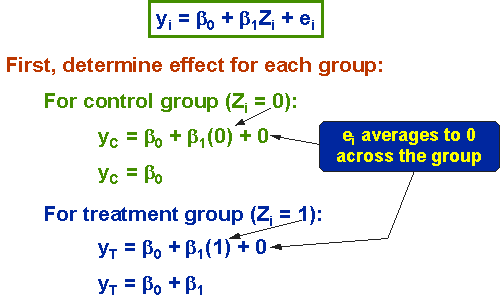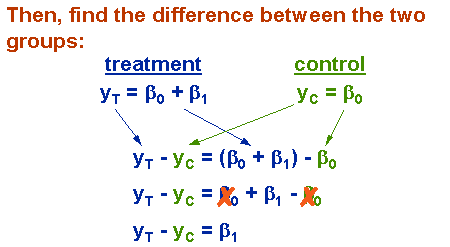Dummy Variables

# Dummy Variables

A dummy variable is a numerical variable used in regression analysis to represent subgroups of the sample in your study. In research design, a dummy variable is often used to distinguish different treatment groups. In the simplest case, we would use a 0,1 dummy variable where a person is given a value of 0 if they are in the control group or a 1 if they are in the treated group. Dummy variables are useful because they enable us to use a single regression equation to represent multiple groups. This means that we don’t need to write out separate equation models for each subgroup. The dummy variables act like ‘switches’ that turn various parameters on and off in an equation. Another advantage of a 0,1 dummy-coded variable is that even though it is a nominal-level variable you can treat it statistically like an interval-level variable (if this made no sense to you, you probably should refresh your memory on levels of measurement). For instance, if you take an average of a 0,1 variable, the result is the proportion of 1s in the distribution.

$$y_i = \beta_0 + \beta_1Z_i +e_i$$

where:

• yi is outcome score of ith unit,

• β0 is coefficient for the intercept,

• β1 is coefficient for the slope,

• Zi is:

• 1 if the ith unit is in the treatment group;
• 0 if the ith unit is in the control group;
• ei is residual for the ith unit.

To illustrate dummy variables, consider the simple regression model for a posttest-only two-group randomized experiment. This model is essentially the same as conducting a t-test on the posttest means for two groups or conducting a one-way Analysis of Variance (ANOVA). The key term in the model is β1, the estimate of the difference between the groups. To see how dummy variables work, we’ll use this simple model to show you how to use them to pull out the separate sub-equations for each subgroup. Then we’ll show how you estimate the difference between the subgroups by subtracting their respective equations. You’ll see that we can pack an enormous amount of information into a single equation using dummy variables. All I want to show you here is that β1 is the difference between the treatment and control groups.

To see this, the first step is to compute what the equation would be for each of our two groups separately. For the control group, Z = 0. When we substitute that into the equation, and recognize that by assumption the error term averages to 0, we find that the predicted value for the control group is β0, the intercept. Now, to figure out the treatment group line, we substitute the value of 1 for Z, again recognizing that by assumption the error term averages to 0. The equation for the treatment group indicates that the treatment group value is the sum of the two beta values.Now, we’re ready to move on to the second step – computing the difference between the groups. How do we determine that? Well, the difference must be the difference between the equations for the two groups that we worked out above. In other word, to find the difference between the groups we just find the difference between the equations for the two groups! It should be obvious from the figure that the difference is β1. Think about what this means. The difference between the groups is β1. OK, one more time just for the sheer heck of it. The difference between the groups in this model is β1!Whenever you have a regression model with dummy variables, you can always see how the variables are being used to represent multiple subgroup equations by following the two steps described above:

• create separate equations for each subgroup by substituting the dummy values
• find the difference between groups by finding the difference between their equations